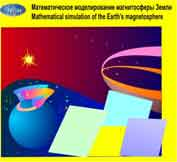ICM SB RAS Russian
 Structure of Institute :: Department of Computational Mathematics
 laboratory grants collaboration employees part time staff papers selected papers about institute researches library feedback contact us метеостанция

# Laboratory 2.2. of Mathematical Models of Near Space

Denisenko Valerij Vasil'evich, Phys.&Math.Dr.

## DescriptionThe main goal of the Laboratory of Mathematical Models for Near-Space is the development of the mathematical model of the magnetosphere of the Earth. The global problem is divided into less complex subproblems and corresponding mathematical models are developed. The magnetohydrodynamical model of the solar wind flow around the magnetospheres of the Earth and other planets, the models of magnetic barrier and dissipative boundary layer are created. Theories of nonlinear and diffusive reconnections of magnetic field of the solar wind with geomagnetic field are developed and are used for calculation of electric fields at the magnetopause. The model of the magnetospheric magnetic field is developed and is used to calculate the electric potential transferring from the magnetopause down to the ionosphere. The model of the ionosphere as a global conductor permits to find the electric fields and currents distributions all over the ionosphere. The high-latitude sources contribution to the electric fields and currents in low-latitude ionosphere are analyzed.

From mathematical point of view all models are the boundary value problems for two- or three-dimensional differential equations that are the results of reducing of magnetohydrodynamical equations using asymptotic small parameters expansions.

Now these submodels are integrating into a few general models, one of which permits to analyze the influence of viscous interaction at the flanks of the magnetospheric tail on the ionospheric electric field. This model includes the following submodels: (a) hypersonic gas overflow around axisymmetrical magnetosphere of given shape, (b) closed magnetospheric magnetic field, (c) magnetic field diffusion through the magnetopause with proper electric field generation, (d) the result normal component of the magnetic filed is put to the model of magnetospheric magnetic filed that is used for mapping, (e) the result electric filed distribution at the magnetopause is mapped into ionospheric cusps and also gives electric potential at the flanks of the plasma sheet, (f) the plasma sheet is simulated as a homogeneous conductor with given velocity distribution, that includes boundary layers at the flanks, (g) the calculated electric field is mapped from the plasma sheet into ionospheric auroral zone, (h) the model of the ionosphere as a global conductor permits to find the electric fields and currents distributions in the rest part of the ionosphere up to the geomagnetic equator.

The development of multigrid method of numerical solution of two-dimensional boundary value problems for elliptic equation with nonsymmetric tensor coefficients is the main laboratory's achievements in the field of numerical mathematics. Symmetrization and variational principles were found and applied to solve these problems.

The designed mathematical methods and software were used for analysis of air pollution propagation, for calculation of magnetic fields in complicated solenoids, for theoretical study of a stationary and oscillatory regimes of lubricated slider bearings, and also for design of technical devises, such as refrigerators and furnaces.